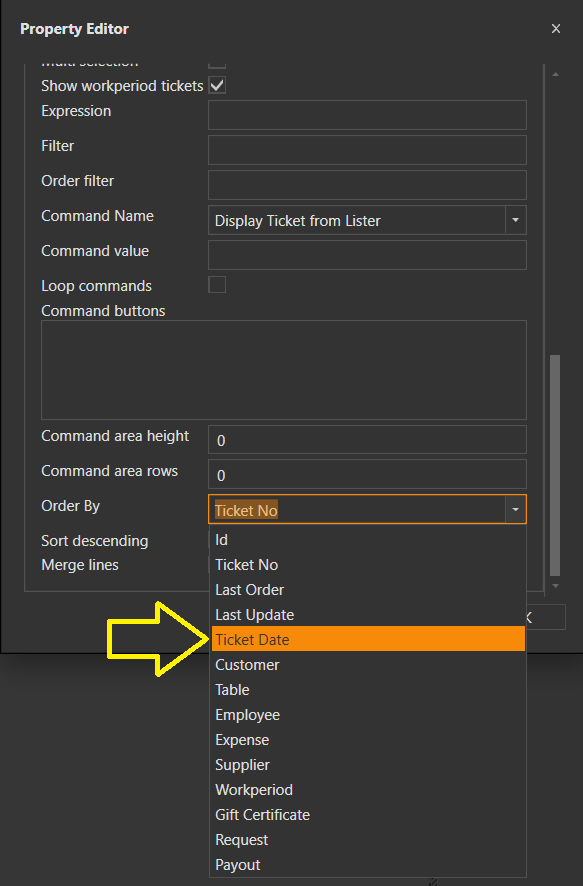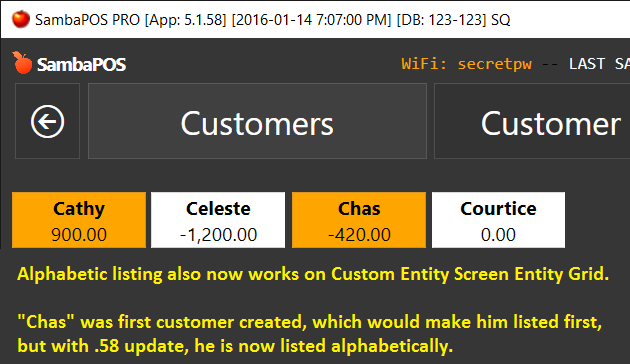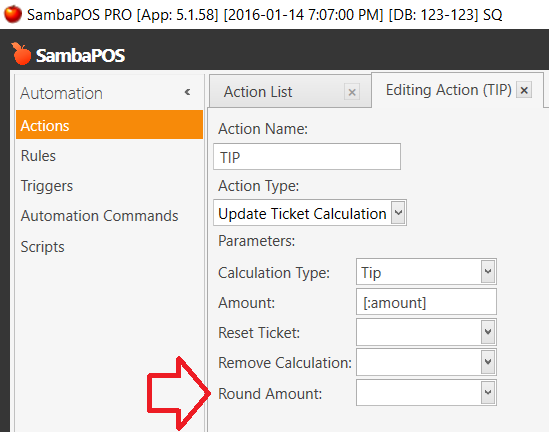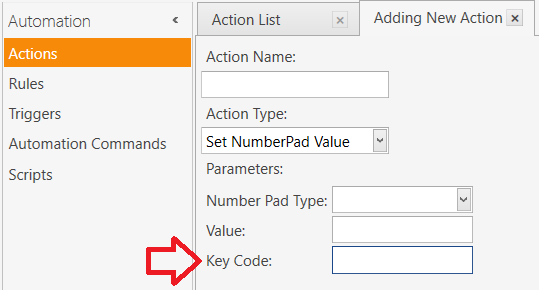# SambaPOS 5.1.58 Releasehttps://sambapos.com/?wpfb_dl=140

New Features & Fixes

• Language Selection during setup will generate default database in that language.
• Using `<img>` tag for Order Tag buttons with Image.
• Retry connecting to database for `Session Provider 19` error.
• Key / Value support for executing “Display Ticket List” action with Ticket Tags.
• Saving Margin Settings for Pivot Table > Print preview window.
• Report explorer date entry fixed
• Unique keys for orders and tickets. Useful for solving “empty key before saving a ticket” related issues .
• Some performance improvements and fine tunes for better usability.

### Order Line Separators

If anyone interested we can add separators between orders.

Update Ticket Line Separator action used for this.

… and `[ORDERS:SEPARATOR]` section used to print separators.

This is an experimental feature though. May not work as expected for all cases we can think of but it should work fine to send additional notes to kitchen.

You can also use `Update Order Separator` action to move order lines under a specific separator.

This is a little tricky as it is hard to determine if we should add a new separator or move order under an existing separator. I mean you should manually handle if you’ll allow separators that have same name. Key parameter does it. If you define a separator by Key (which can be obtained by `{SEPARATOR KEY}` it will use existing separator. If defined by Name and if it is a new order it will again use existing separator. Well hard to explain but you’ll get the idea when you try that with a specific use case.

###Implemented: Ticket Lister - sort by Date###Implemented - list Entities alphabetically:###Implemented - Update Ticket Calculation Action has Round function:###Implemented - Entity Custom Data fields in Report tags:

``````[Sales:2,2,2, 1]
{REPORT TICKET DETAILS:T.TicketNumber,EN.Deliverer,EN.Bairro,EC.Bairros.Taxa:(TEN.Deliverer=Diogo MB)}
>Total
``````

###Implemented - `Key Code` Parameter added to `Set NumberPad Value` Action

The `Key Code` Parameter allows us to send special keyboard codes via this action.``````LBUTTON = 1,
RBUTTON = 2,
CANCEL = 3,
MBUTTON = 4,
XBUTTON1 = 5,
XBUTTON2 = 6,
BACK = 8,
TAB = 9,
CLEAR = 12,
RETURN = 13,
SHIFT = 16,
CONTROL = 17,
PAUSE = 19,
CAPITAL = 20,
KANA = 21,
HANGEUL = 21,
HANGUL = 21,
JUNJA = 23,
FINAL = 24,
HANJA = 25,
KANJI = 25,
ESCAPE = 27,
CONVERT = 28,
NONCONVERT = 29,
ACCEPT = 30,
MODECHANGE = 31,
SPACE = 32,
PRIOR = 33,
NEXT = 34,
END = 35,
HOME = 36,
LEFT = 37,
UP = 38,
RIGHT = 39,
DOWN = 40,
SELECT = 41,
PRINT = 42,
EXECUTE = 43,
SNAPSHOT = 44,
INSERT = 45,
DELETE = 46,
HELP = 47,
VK_0 = 48,
VK_1 = 49,
VK_2 = 50,
VK_3 = 51,
VK_4 = 52,
VK_5 = 53,
VK_6 = 54,
VK_7 = 55,
VK_8 = 56,
VK_9 = 57,
VK_A = 65,
VK_B = 66,
VK_C = 67,
VK_D = 68,
VK_E = 69,
VK_F = 70,
VK_G = 71,
VK_H = 72,
VK_I = 73,
VK_J = 74,
VK_K = 75,
VK_L = 76,
VK_M = 77,
VK_N = 78,
VK_O = 79,
VK_P = 80,
VK_Q = 81,
VK_R = 82,
VK_S = 83,
VK_T = 84,
VK_U = 85,
VK_V = 86,
VK_W = 87,
VK_X = 88,
VK_Y = 89,
VK_Z = 90,
LWIN = 91,
RWIN = 92,
APPS = 93,
SLEEP = 95,
MULTIPLY = 106,
SEPARATOR = 108,
SUBTRACT = 109,
DECIMAL = 110,
DIVIDE = 111,
F1 = 112,
F2 = 113,
F3 = 114,
F4 = 115,
F5 = 116,
F6 = 117,
F7 = 118,
F8 = 119,
F9 = 120,
F10 = 121,
F11 = 122,
F12 = 123,
F13 = 124,
F14 = 125,
F15 = 126,
F16 = 127,
F17 = 128,
F18 = 129,
F19 = 130,
F20 = 131,
F21 = 132,
F22 = 133,
F23 = 134,
F24 = 135,
NUMLOCK = 144,
SCROLL = 145,
LSHIFT = 160,
RSHIFT = 161,
LCONTROL = 162,
RCONTROL = 163,
BROWSER_BACK = 166,
BROWSER_FORWARD = 167,
BROWSER_REFRESH = 168,
BROWSER_STOP = 169,
BROWSER_SEARCH = 170,
BROWSER_FAVORITES = 171,
BROWSER_HOME = 172,
VOLUME_MUTE = 173,
VOLUME_DOWN = 174,
VOLUME_UP = 175,
MEDIA_NEXT_TRACK = 176,
MEDIA_PREV_TRACK = 177,
MEDIA_STOP = 178,
MEDIA_PLAY_PAUSE = 179,
LAUNCH_MAIL = 180,
LAUNCH_MEDIA_SELECT = 181,
LAUNCH_APP1 = 182,
LAUNCH_APP2 = 183,
OEM_1 = 186,
OEM_PLUS = 187,
OEM_COMMA = 188,
OEM_MINUS = 189,
OEM_PERIOD = 190,
OEM_2 = 191,
OEM_3 = 192,
OEM_4 = 219,
OEM_5 = 220,
OEM_6 = 221,
OEM_7 = 222,
OEM_8 = 223,
OEM_102 = 226,
PROCESSKEY = 229,
PACKET = 231,
ATTN = 246,
CRSEL = 247,
EXSEL = 248,
EREOF = 249,
PLAY = 250,
ZOOM = 251,
NONAME = 252,
PA1 = 253,
OEM_CLEAR = 254
``````
6 Likes

@emre what do you need from us to add other languages?

This topic was automatically closed 2 days after the last reply. New replies are no longer allowed.